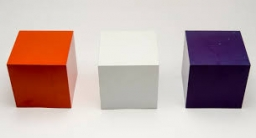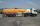# The volume 2

The volume of a cube is 27 cubic meters. Find the height of the cube.

h =  3 m

### Step-by-step explanation:Did you find an error or inaccuracy? Feel free to write us. Thank you!Tips to related online calculators
Tip: Our volume units converter will help you with the conversion of volume units.

## Related math problems and questions:

• Area of a cubeCalculate the surface area of a cube if its volume is equal to 729 cubic meters.
• Cube V2SThe volume of the cube is 27 dm cubic. Calculate the surface of the cube.
• Cube surface and volumeFind the surface of the cube with a volume of 27 dm3.
• Cube diagonalsCalculate the length of the side and the diagonals of the cube with a volume of 27 cm3.
• Equilateral cylinderEquilateral cylinder (height = base diameter; h = 2r) has a volume of V = 199 cm3 . Calculate the surface area of the cylinder.
• Cube 9What was the cube's original edge length after cutting 39 small cubes with an edge of 2 dm left 200 dm3?
• The cubeThe cube has a surface area of 216 dm2. Calculate: a) the content of one wall, b) edge length, c) cube volume.
• Body diagonalCalculate the cube volume, whose body diagonal size is 75 dm. Draw a picture and highlight the body diagonal.
• Cube basicsHow long is the edge length of a cube with volume 15 m3?
• Cube surface and volumeThe surface of the cube is 500 cm2, how much cm3 will be its volume?
• Cube surfce2volumeCalculate the volume of the cube if its surface is 150 cm2.
• Sphere A2VThe surface of the sphere is 241 mm2. What is its volume?
• Gasoline tank cylindricalWhat is the inner diameter of the tank, which is 8 m long and contains 40 cubic cubic meters of gasoline?
• Volume and areaWhat is the volume of a cube which has an area of 361 cm2?
• Tetrahedral prismThe height of a regular tetrahedral prism is three times greater than the length of the base edge. Calculate the length of the base edge, if you know that the prism volume is 2187 cm3.
• The cubeThe cube has a surface area of 486 m ^ 2. Calculate its volume.
• Length of the edgeFind the length of the edge of a cube with a cm2 surface and a volume in cm3 expressed by the same number.